## Series Solutions: IntroductionThroughout these pages I will assume that you are familiar with power series and the concept of the radius of convergence of a power series.

Let us consider the example of the second order differential equation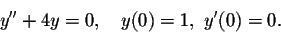You probably already know that the solution is given by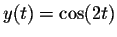; recall that this function has as its Taylor series

cos(2t) = 1 -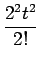+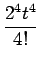-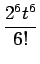+...,

and that this representation is valid for all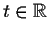.

Do you remember how to compute the Taylor series expansion for a given function?

If everything works out, then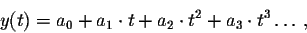where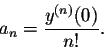Here y(n)(0) denotes the nth derivative of y at the point t=0.

What does this mean? To compute the Taylor series for the solution to our differential equation, we just have to compute its derivatives. Note that the initial conditions give us a head start: y(0)=1, so a0=1. y'(0)=0, so a1=0.

We can rewrite the differential equation as

y''=-4y,

so in particular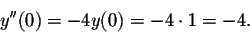Consequently a2=-4/2!=-2. By now, we have figured out that the solution to our differential equation has as its second degree Taylor polynomial the function 1-2t2.

Next we differentiate

y''=-4y

on both sides with respect to t, to obtain

y'''=-4y',

so in particular

y'''(0)=-4y'(0)=0,

yielding a3=0.

We can continue in this fashion as long as we like: Differentiating

y'''=-4y'

yields

y(4)=-4y'',

in particular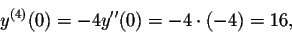so a4=16/4!=2/3. At this point we have figured out that the solution to our differential equation has as its fourth degree Taylor polynomial the function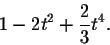We expect that this Taylor polynomial is reasonably close to the solution y(t) of the differential equation, at least close to t=0. In the picture below, the solutionis drawn in red, while the power series approximation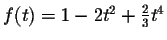is depicted in blue: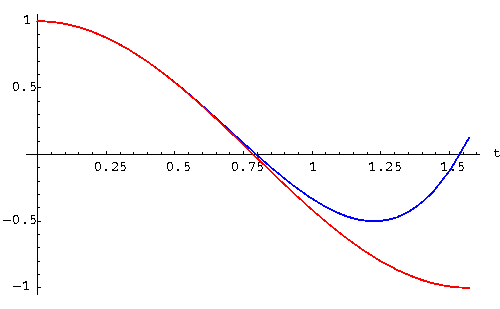The method outlined works also in theory for non-linear differential equations, even though the computational effort usually becomes prohibitive after the first few steps. Let's consider the example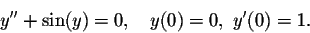We try to find the Taylor polynomials for the solution, of the form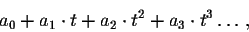whereThe initial conditions yield a0=0 and a1=1. To find a2, we rewrite the differential equation as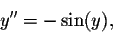and plug in t=0: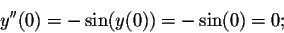consequently a2=0.

Next we differentiate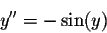on both sides with respect to t, to obtain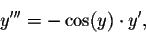so in particular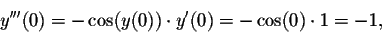yielding a3=-1/3!=-1/6. Note that since we were differentiating with respect to t, we had to use the chain rule to find the derivative of the term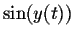.

Let's continue a little bit longer: We differentiateto obtain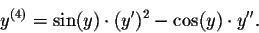Consequently y(4)(0)=0, and thus a4=0.

Differentiating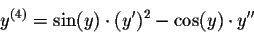yields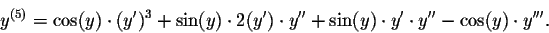You can check that y(5)=y'(0)3-y'''(0)=1-(-1)=2 and thus a5=2/5!.

The fifth degree Taylor polynomial approximation to the solution of our differential equation has the form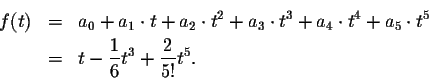We again expect that this Taylor polynomial is reasonably close to the solution y(t) of the differential equation, at least close to t=0. In the picture below, the solution, as computed by a numerical method, is drawn in red, while the power series approximation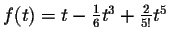is depicted in blue: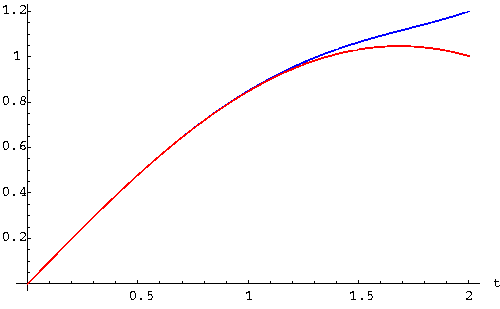The next sections will develop an organized method to find power series solutions for second order linear differential equations. Here are a couple of examples to practice what you have learned so far:

#### Exercise 1:

Find the fifth degree Taylor polynomial of the solution to the differential equation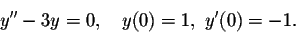#### Exercise 2:

Find the fourth degree Taylor polynomial of the solution to the differential equation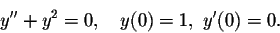[Back] [Next]
[Algebra] [Trigonometry] [Complex Variables]
[Calculus] [Differential Equations] [Matrix Algebra]S.O.S MATHematics home page

Do you need more help? Please post your question on our S.O.S. Mathematics CyberBoard.Helmut Knaust
1998-06-29